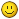# Linear gamma

• crabshank
•Topic Author
• Offline
1 year 2 months ago - 3 months 11 hours ago #1 by crabshank
Linear gamma was created by crabshank
A very simple shader that reverse and applies transfer functions:
```#include "ReShadeUI.fxh"

uniform int Gamma_type < __UNIFORM_COMBO_INT1
ui_items = "sRGB -> linear RGB\0linear RGB -> sRGB\0Rec.(2020/601/709) RGB -> linear RGB\0linear RGB -> Rec.(2020/601/709) RGB\0gamma=2.2 -> linear RGB\0linear RGB -> gamma=2.2\0gamma=2.6 -> linear RGB\0linear RGB -> gamma=2.6\0";
> = 0;

uniform int Gamma_type_2 < __UNIFORM_COMBO_INT1
ui_items = "sRGB -> linear RGB\0linear RGB -> sRGB\0Rec.(2020/601/709) RGB -> linear RGB\0linear RGB -> Rec.(2020/601/709) RGB\0gamma=2.2 -> linear RGB\0linear RGB -> gamma=2.2\0gamma=2.6 -> linear RGB\0linear RGB -> gamma=2.6\0";
ui_tooltip = "Transfer function for the second instance of the shader, if enabled.";
> = 1;

#include "DrawText_mod.fxh"

#define rcpTwoFour 1.0/2.4
#define rcpOFiveFive 1.0/1.055
#define rcpTwelveNineTwo 1.0/12.92
#define recAlpha 1.09929682680944
#define rcpRecAlpha 1.0/1.09929682680944
#define recBeta 0.018053968510807
#define recBetaLin 0.004011993002402
#define rcpFourFive 1.0/4.5
#define rcpTxFourFive 10.0/4.5
#define invTwoTwo 5.0/11.0
#define invTwoSix 5.0/13.0

float4 LinearGammaPass(float4 position : SV_Position, float2 texcoord : TEXCOORD0) : SV_Target
{

float4 c1 = c0;

[branch]if (Gamma_type==0){ //sRGB transfer
c1.rgb=(c0.rgb> 0.00313066844250063)?1.055 * pow(c0.rgb,rcpTwoFour) - 0.055:12.92 *c0.rgb;
}else if(Gamma_type==6){
c1.rgb=pow(c0.rgb,invTwoSix);
}else if (Gamma_type==4){
c1.rgb=pow(c0.rgb,invTwoTwo);
}else if(Gamma_type==2){
c1.rgb=(c0.rgb< recBeta)?4.5*c0.rgb:recAlpha*pow(c0.rgb,0.45)-(recAlpha-1);
}else if(Gamma_type==1){
c1.rgb=(c0.rgb > 0.0404482362771082 )?pow(abs((c0.rgb+0.055)*rcpOFiveFive),2.4):c0.rgb*rcpTwelveNineTwo;
}else if(Gamma_type==7){
c1.rgb=pow(c0.rgb,2.6);
}else if(Gamma_type==5){
c1.rgb=pow(c0.rgb,2.2);
}else if(Gamma_type==3){
c1.rgb=(c0.rgb < recBetaLin )?rcpFourFive*c0.rgb:pow(-1*(rcpRecAlpha*(1-recAlpha-c0.rgb)),rcpTxFourFive);
}

return c1;

}

float4 LinearGammaPass2(float4 position : SV_Position, float2 texcoord : TEXCOORD0) : SV_Target
{

float4 c1 = c0;

[branch]if (Gamma_type_2==0){ //sRGB transfer
c1.rgb=(c0.rgb> 0.00313066844250063)?1.055 * pow(c0.rgb,rcpTwoFour) - 0.055:12.92 *c0.rgb;
}else if(Gamma_type_2==6){
c1.rgb=pow(c0.rgb,invTwoSix);
}else if (Gamma_type_2==4){
c1.rgb=pow(c0.rgb,invTwoTwo);
}else if(Gamma_type_2==2){
c1.rgb=(c0.rgb< recBeta)?4.5*c0.rgb:recAlpha*pow(c0.rgb,0.45)-(recAlpha-1);
}else if(Gamma_type_2==1){
c1.rgb=(c0.rgb > 0.0404482362771082 )?pow(abs((c0.rgb+0.055)*rcpOFiveFive),2.4):c0.rgb*rcpTwelveNineTwo;
}else if(Gamma_type_2==7){
c1.rgb=pow(c0.rgb,2.6);
}else if(Gamma_type_2==5){
c1.rgb=pow(c0.rgb,2.2);
}else if(Gamma_type_2==3){
c1.rgb=(c0.rgb < recBetaLin )?rcpFourFive*c0.rgb:pow(-1*(rcpRecAlpha*(1-recAlpha-c0.rgb)),rcpTxFourFive);
}

return c1;

}

technique Linear_Gamma
{
pass
{
}
}

technique Linear_Gamma_2
{
pass
{
}
}```

Then re-grade as you see fit, I suggest applying gamma (choosing your own value or algorithm could lead to better results) first.

The image above uses the inverse (sRGB) setting.

I also have a version that uses an implicit method, but the gamma function is less accurate (straight gamma of 2.2) here
Last edit: 3 months 11 hours ago by crabshank. Reason: Updated
The following user(s) said Thank You: Wicked Sick, sajittarius, jas01, Deathmedic, Cul

• crabshank
•Topic Author
• Offline
1 year 1 week ago #2 by crabshank
Replied by crabshank on topic sRGB to Linear RGB (approximation)
UPDATE: Included both transforms (to Linear and inverse).

• sajittarius
•• Offline
1 year 1 week ago #3 by sajittarius
Replied by sajittarius on topic sRGB to Linear RGB (approximation)
Would it be possible to make a version of this with an adjustable gamma slider? I tried it in Star Wars Jedi: Fallen Order with HDR on and its amazing but could use a tiny tweak to the gamma values.

This shader works in HDR, whilst many other gamma ones (LevelsPlus, Tonemap, etc) do not, so i could see it helping in a lot of games where the HDR seems washed out.
The following user(s) said Thank You: crabshank

• crabshank
•Topic Author
• Offline
1 year 1 week ago #4 by crabshank
Replied by crabshank on topic sRGB to Linear RGB (approximation)
UPDATE: Separated transforms into separate techniques.

@sajittarius using my grey gamma shader after this should help.
The following user(s) said Thank You: sajittarius

• sajittarius
•• Offline
1 year 6 days ago #5 by sajittarius
Replied by sajittarius on topic sRGB to Linear RGB (approximation)
i think the grey gamma shader was what i was looking for in the first place (it works great for what i want just by tweaking that shader)

thank you• crabshank
•Topic Author
• Offline
3 months 2 days ago #6 by crabshank
Replied by crabshank on topic Linear gamma
I have included more transfer functions in this shader, check my other shaders as I have added many more colour spaces to most of them.

•Topic Author